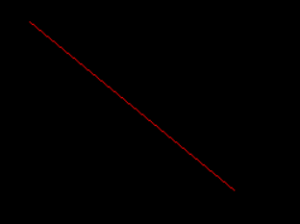# Python PIL | ImageDraw.Draw.line()

PIL is the Python Imaging Library which provides the python interpreter with image editing capabilities. The `ImageDraw` module provide simple 2D graphics for Image objects. You can use this module to create new images, annotate or retouch existing images, and to generate graphics on the fly for web use.

`ImageDraw.Draw.line()` Draws a line between the coordinates in the xy list.

Syntax: PIL.ImageDraw.Draw.line(xy, fill=None, width=0)

Parameters:
xy – Sequence of either 2-tuples like [(x, y), (x, y), …] or numeric values like [x, y, x, y, …].
fill – Color to use for the line.
width –The line width, in pixels. Note that line joins are not handled well, so wide polylines will not look good.

Returns: An Image object in ellipse shape.

 `  `  ` `  `# importing image object from PIL ` `import` `math ` `from` `PIL ``import` `Image, ImageDraw ` ` `  `w, h ``=` `220``, ``190` `shape ``=` `[(``40``, ``40``), (w ``-` `10``, h ``-` `10``)] ` ` `  `# creating new Image object ` `img ``=` `Image.new(``"RGB"``, (w, h)) ` ` `  `# create line image ` `img1 ``=` `ImageDraw.Draw(img)   ` `img1.line(shape, fill ``=``"none"``, width ``=` `0``) ` `img.show() `Another Example: Here we use different colour for filling.

 `  `  ` `  `# importing image object from PIL ` `import` `math ` `from` `PIL ``import` `Image, ImageDraw ` ` `  `w, h ``=` `220``, ``190` `shape ``=` `[(``40``, ``40``), (w ``-` `10``, h ``-` `10``)] ` ` `  `# creating new Image object ` `img ``=` `Image.new(``"RGB"``, (w, h)) ` ` `  `# create line image ` `img1 ``=` `ImageDraw.Draw(img)   ` `img1.line(shape, fill ``=``"red"``, width ``=` `0``) ` `img.show() `

Output:My Personal Notes arrow_drop_upCheck out this Author's contributed articles.

If you like GeeksforGeeks and would like to contribute, you can also write an article using contribute.geeksforgeeks.org or mail your article to contribute@geeksforgeeks.org. See your article appearing on the GeeksforGeeks main page and help other Geeks.

Please Improve this article if you find anything incorrect by clicking on the "Improve Article" button below.

Article Tags :

Be the First to upvote.

Please write to us at contribute@geeksforgeeks.org to report any issue with the above content.